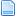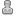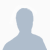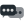Hello Guest it is April 12, 2021, 05:56:49 PM

###Author Topic: Little Number Macro  (Read 1348 times)

0 Members and 1 Guest are viewing this topic.

####ozwes007•103##### Little Number Macro
« on: June 22, 2015, 04:03:38 AM »
Hi Guys and Girls,

Just a little number Macro that I used to find an error that was creeping into my Maths while using Mach3.
It shows the difference between different types of Numbers.
To view some of the more exotic things try numbers like 35649879.654987243553

Macro - Just cut and paste the below code into the VB editor in Mach3 and save as Maths.m1s or whatever you like ;-)

Sub Main
Dim Msg, X, Y

X = InputBox("Enter a Number")

Y = Abs(X)
Msg = "The Absolute Value[Abs(X)] is " & Y
MsgBox Msg,64,"The Absolute Value of " & X  'Display Message

Y = Round(X,2)
Msg = "The Rounded Value[2 decimal places] is " & Y
MsgBox Msg,64,"The Rounding Value of " & X  'Display Message

Y = Format(X,"00##.###000000")
Msg = "The Format Value[00##.###00000] is " & Y
MsgBox Msg,64,"How to Format the Value of " & X  'Display Message

Y = Format(X,"Fixed")
Msg = "The Format Value[Fixed] is " & Y
MsgBox Msg,64,"The Formatted Fixed Value of " & X  'Display Message

Y = Format(X,"General Number")
Msg = "The Format Value[General Number] is " & Y
MsgBox Msg, 64,"The General Number Value of " & X  'Display Message

Y = Format(X,"Standard")
Msg = "The Format Value[Standard] is " & Y
MsgBox Msg,64,"The Standard Value of " & X  'Display Message

Y = Format(X,"Percent")
Msg = "The Format Value[Percent] is " & Y
MsgBox Msg,64,"The Percentage Value of " & X  'Display Message

Y = Fix(X)
Msg = "The Fixed Value[Fix(X)] is " & Y
MsgBox Msg,64,"The Fixed Value of " & X  'Display Message

Y = Hex(X)
Msg = "The Hexidecimal Value[Hex(X)] is " & Y
MsgBox Msg,64,"The Hexidecimal Value of " & X  'Display Message

Y = Oct(X)
Msg = "The Octagonal Value[Oct(X)] is " & Y
MsgBox Msg,64,"The Octagonal Value of " & X  'Display Message

Y = Int(X)
Msg = "The Interger Value[Int(X)] is " & Y
MsgBox Msg, 64,"The Integer of " & X  'Display Message

Y = Log(X)
Msg = "The Natural Log Value[Log(X)] is " & Y
MsgBox Msg,64,"The Log of " & X  'Display Message

Y = Sqr(X)
Msg = "The Square Root Value[Sqr(X)] is " & Y
MsgBox Msg,64,"The Square Root of " & X  'Display Message

Y = Val(X)
Msg = "The Numeric Value[Val(X)] is " & Y
MsgBox Msg,64,"The Value of " & X  'Display Message

End Sub

Wes